• 随机过程中 由初始分布和一步转移矩阵 得到最终的状态 由Matlab程序可直接运行 能够成功运行的
• 最近在学习卡尔曼滤波需要在matlab/simulink系统中将连续系统进行离散化 ... 数学过程推导 具'j体的数i学推导过程a就不做详绍了，在现代控制理论教材中都有，...求系统的状态转移矩阵,式中为拉氏反变换 matlab求解过.
最近在学习卡尔曼滤波需要在matlab/simulink系统中将连续系统进行离散化
推荐一篇个人觉得不错的卡尔曼滤波原理详解https://blog.csdn.net/weixin_43942325/article/details/103416681
数学过程推导
具'j体的数i学推导过程a就不做详绍了，在现代控制理论教材中都有，这儿贴两张图片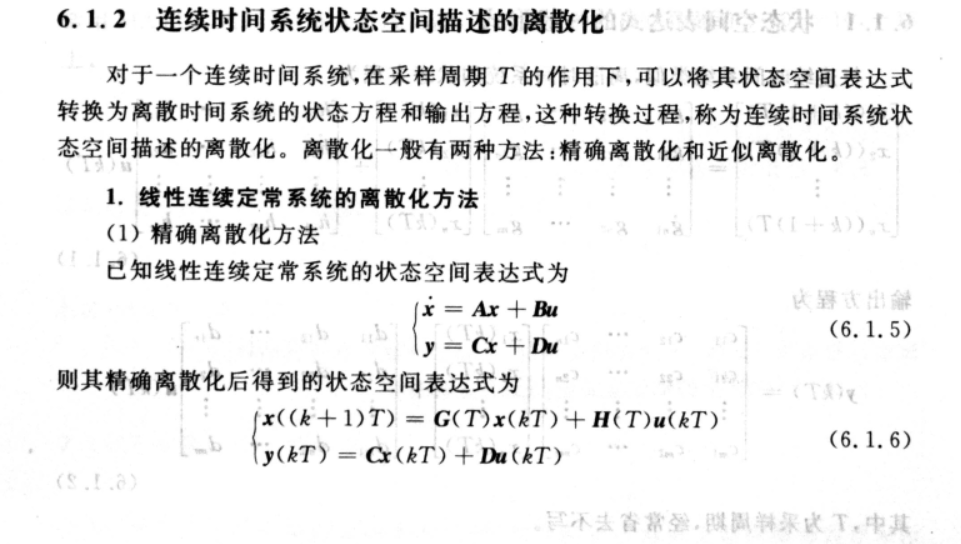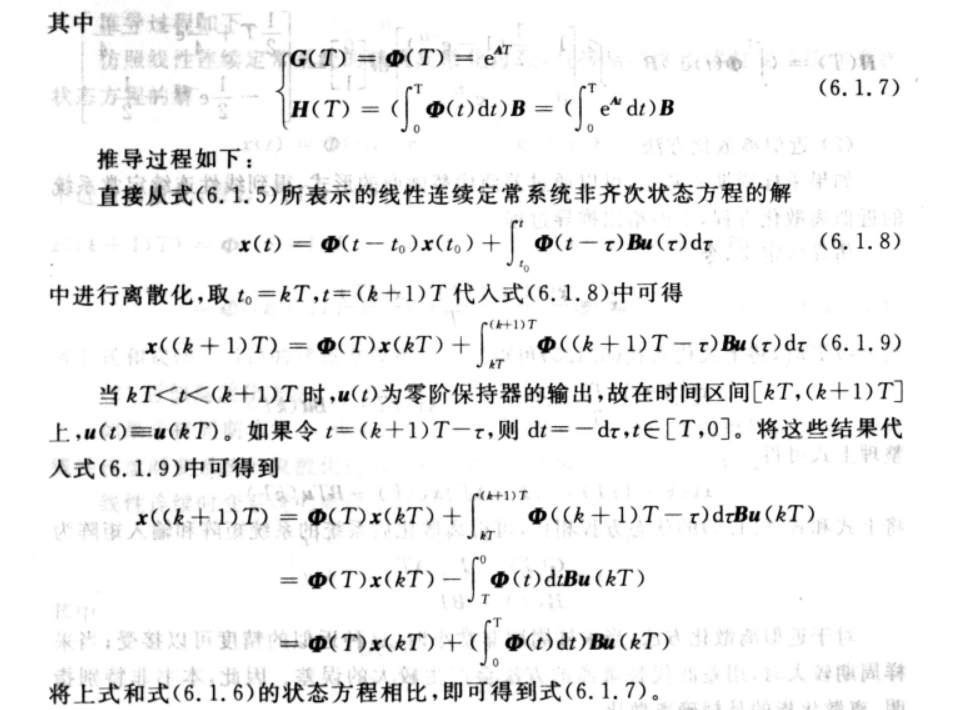matlab 程序编写
根据上面公式推导，假设存在系统,其中,
其离散系统表达式为
求系统的状态转移矩阵,式中为拉氏反变换
matlab求解过程为
A = [0 1;0 -2];
B1 = [0 ;1];
syms s t Ls;   % 求状态转移矩阵 利用拉氏变换，syms为符号函数用来定义数学函数
I = eye(size(A));
Ls = inv(s*I - A);
STM = ilaplace(Ls,s,t) %状态转移矩阵,ilaplace为拉氏反变换函数

同理可以求H
syms s t Ls;   % 求状态转移矩阵 利用拉氏变换
I = eye(size(A));
Ls = inv(s*I - A);% collect 函数为合并同类项
STM = ilaplace(Ls,s,t) %状态转移矩阵

syms T
HLs = int(STM,t,0,T);
H = HLs*B1
运行结果如下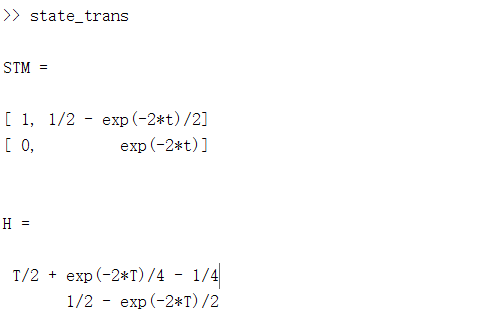dt = 0.001;
A = [0 1;0 -2];
B1 = [0 ;1];
syms s t Ls;   % 求状态转移矩阵 利用拉氏变换
I = eye(size(A));
Ls = inv(s*I - A);% collect 函数为合并同类项
STM = ilaplace(Ls,s,t); %状态转移矩阵
G = double(subs(STM,t,dt)) % 符号函数求解
syms T
HLs = int(STM,t,0,T);
H = HLs*B1;
H = real(double(subs(H,T,dt))) % 符号函数求解
结果如下：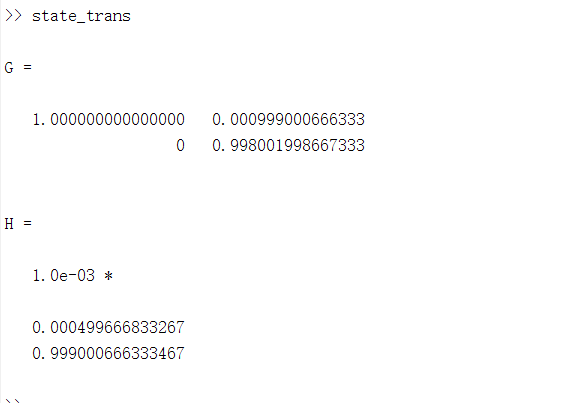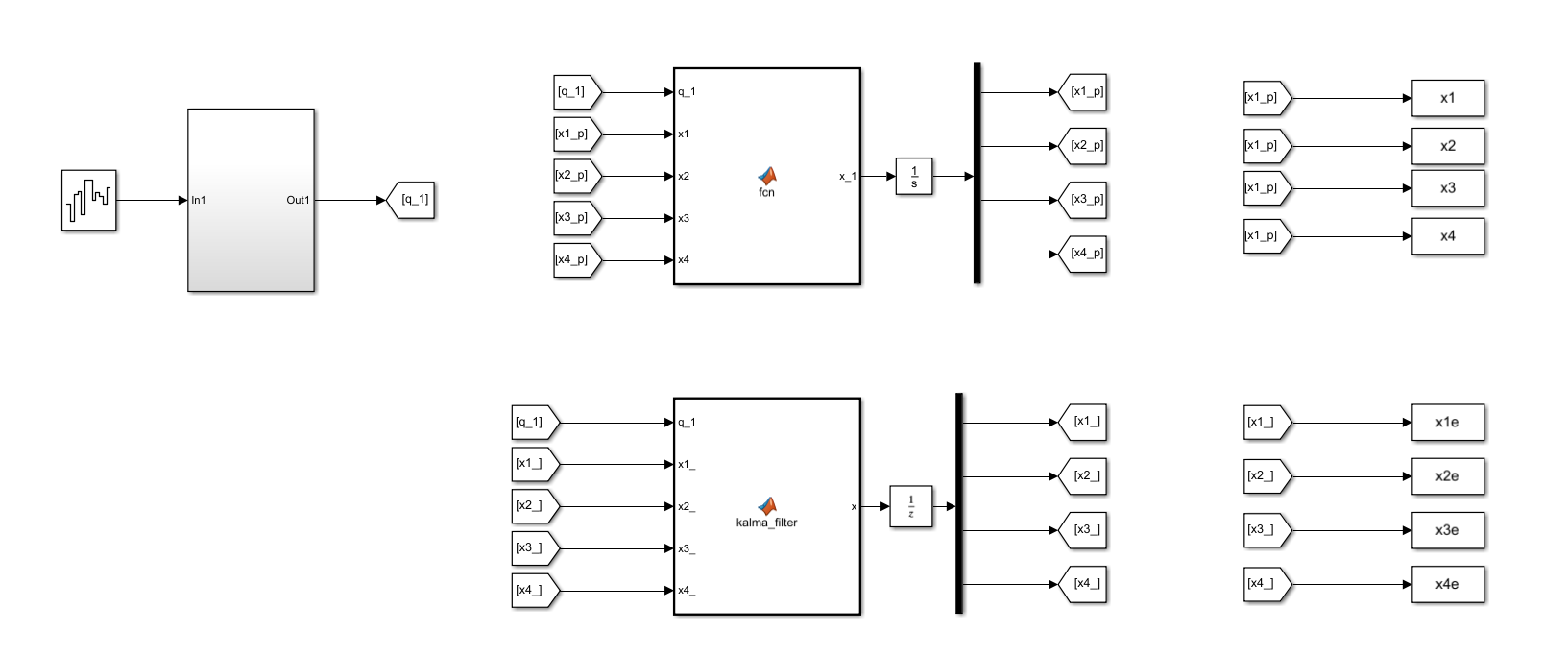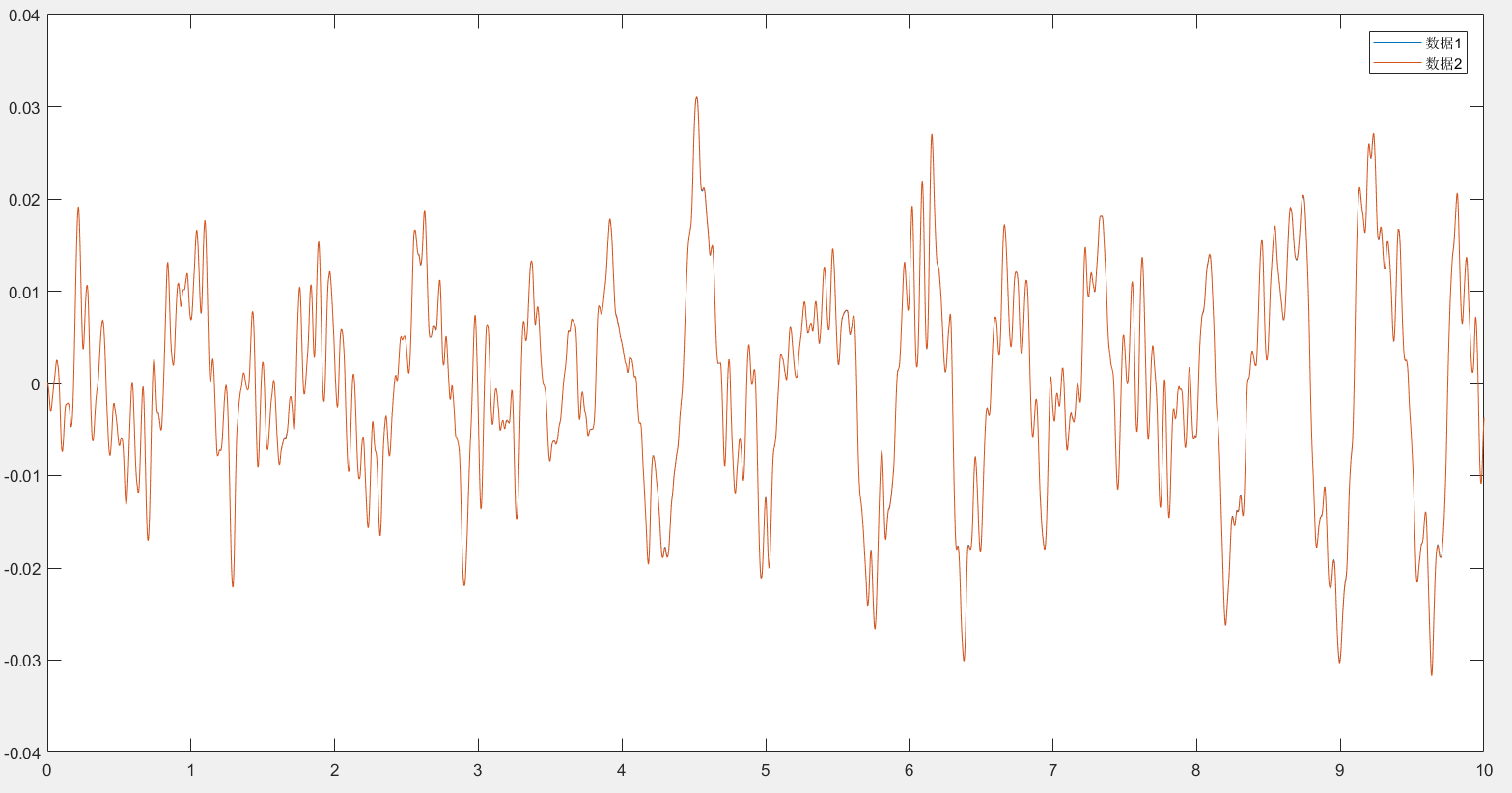展开全文matlab 算法 傅立叶分析
• 泻药，根据已有数据得到统计上的转移矩阵常用的方法有两种一下例子是信用风险管理的例子，但是统计手段是通用的：Cohort approach：对于特处在给定状态i下的观测目标”，在给定一个观测期里转移到状态j的“概率”...


泻药，根据已有数据得到统计上的转移矩阵常用的方法有两种一下例子是信用风险管理的例子，但是统计手段是通用的：
Cohort approach：对于特处在给定状态i下的观测目标”，在给定一个观测期里转移到状态j的“概率”（这个是我们观测的）为：（1）其中N为观测的数量
那么对于所有观测目标而言，我们要对所有观测期内的转移概率进行加权（2）
对所有状态组合i，j（假如转移的两个方向状态数对等，总共有n个，那么应该是n^2个状态组合，所以矩阵也是个方阵）
这个方法有个缺陷，依赖于观测时间段。如果不标准化观测期，观测时间段不一，得出的结论很可能有误差
Harzard rate approach：这个方法叫“密度法”，核心思想是假设事件转移是个markov chain。我们只需求出一个”单位转移密度矩阵“就可以生成所有时间里的转移矩阵
比如状态i转移到状态j的密度可以表示为：（3）我们以delta s 为时间间隔，记录0 到 T每一个时间段还是i状态的目标的数量。得到了一个不依赖于时间的“单位时间转移矩阵”再另对角线上的元素为：（4）
当delta s非常小的时候，式（3）的分母可以近似为积分，在Markov chain中，转移矩阵的连乘形式也相应的近似成指数形式(泰勒展开）：（5）式（5）大概就是题主所说的“时间的函数”


展开全文• 将时间分片后打算将图一转换为图二的效果，即将每个人的一天的状态按一天144个10分钟去分成144列 注意：某些人的某个时间段可能会有多个活动，因为某些活动少于10分钟的原因 图一 图二 ​​​​ 代码如图：...
 承接上文：pandas：参照列属性将一行转换为多行-时间精度分片
将时间分片后打算将图一转换为图二的效果，即将每个人的一天的状态按一天144个10分钟去分成144列
注意：某些人的某个时间段可能会有多个活动，因为某些活动少于10分钟的原因

图一

图二
​​​​

代码如图：（可处理出图二）
#将活动表按照144个10分钟分组，活动类型按"|"划分
dt = DataTable.groupby(['TUCASEID','TUSTARTTIM']).apply(lambda x:['|'.join(x['state'])])
#Seriers转dataframe
dt2= dt.to_frame()
dt2.rename(columns={0:'STATE'},inplace=True)
#字符串相加为列表
dt3 = dt.groupby(['TUCASEID']).sum()
#Seriers转dataframe,的到每个ID的一行（一行有一个144的list）
dt4 = dt3.to_frame()
dt4.rename(columns={0:'STATE'},inplace=True)
#list转str然后去除'[]'
dt4['STATE'] = dt4['STATE'].apply(lambda x:str(x).strip('[]'))
#按，分割成多列（outer以防万一）
dt4 = dt4['STATE'].str.split(',',expand=True)
#去除左右空格
dt4 = dt4.applymap(lambda x: str(x).strip())
dt4.head()
关键在于groupby的用法，groupby使你能以一种自然的方式对数据集进行切片、切块、摘要等操作。根据一个或多个键（可以是函数、数组或DataFrame列名）拆分pandas对象。
操作groupby后，groupby括号后里的属性不会重复（可以组合索引）。
groupby后可以使用系统函数sum(),mean()等，也可以使用自定义函数去对数据分组。

得到状态转移矩阵：
#获取第Index个十分钟到第Index+1个分钟的状态转移矩阵
def GetCounts(Index):
TimeRow = dt4.iloc[:,Index:Index+2]
Act_list = ['Sleep','HouseAct','WithChild','Work','Educaton','Consumer','L.S.R','Other']
#总人数1
test_sum = 0
for x in Act_list:
test_sum+= len(TimeRow[TimeRow[Index].str.contains(x)])

#总人数2
peo_sum = 0
for x in Act_list:
for y in Act_list:
peo_sum+= len(TimeRow[(TimeRow[Index].str.contains(x)) & (TimeRow[Index+1]).str.contains(y)])

Big_Temp_list = list()
for x in Act_list:
Temp_list = list()
testpeonum = len(TimeRow[TimeRow[Index].str.contains(x)])
#每个状态的比例
testpro = testpeonum/test_sum

for y in Act_list:
peonum = len(TimeRow[(TimeRow[Index].str.contains(x)) & (TimeRow[Index+1]).str.contains(y)])
#状态一到状态二的概率
Proportion = peonum / peo_sum if peonum != 0 else 0
#状态一到状态二的概率 / 状态一的概率
finalpro = Proportion / testpro if Proportion != 0 else 0
Temp_list.append(finalpro)
Big_Temp_list.append(Temp_list)

#STARTTIME = datetime.datetime.strptime('04:00:00','%H:%M:%S') + datetime.timedelta(minutes = Index * 10)
#ENDTIME = datetime.datetime.strptime('04:00:00','%H:%M:%S') + datetime.timedelta(minutes = (Index+1) * 10)
#STARTTIME = STARTTIME.strftime('%H:%M:%S')
#ENDTIME = ENDTIME.strftime('%H:%M:%S')
#print('时刻： '+STARTTIME+'  TO  '+ENDTIME)
Matrix = np.array(Big_Temp_list)
return Matrix
可以看出使用两个循环可以获得一个8*8的状态转移矩阵
至于如何计算状态转移矩阵：
下面介绍一步转移概率矩阵的实现方法：
1.首先计算每一种状态的概率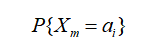2.计算m时刻状态为ai,m+1时刻状态为aj的概率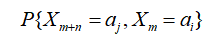3.计算转移概率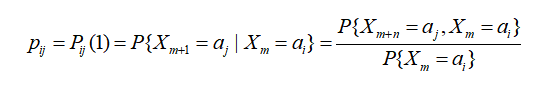举个例子，有一个序列X= {a,c,a,c,a,b,d,b};
1.首先它的状态空间为I = {a,b,c,d},计算每一种状态的概率 p(a) = 3/8,p(b) = 2/8,p(c) = 2/8,p(d) = 1/8;
2.计算m时刻状态为ai且m+1时刻状态为aj的概率，比如，计算当前时刻为a下一时刻为c的转移概率
由于举的例子状态数比较少很容易知道 p = 1/4;
3.经过上面的计算就可以得出转移概率p(a->c) = p/p(a) = 2/3.
如果上面的过程不好理解，可以用古典概型来计算，为了求p(a->c)可以分别求出当前状态为a时
下一状态为b、c、d的次数：
a->a 0次； a->b 1次；a->c 2次；a->d 0次；
p(a->c)=2/(0+1+2+0)=2/3
为了更好的理解，下面给出序列X的一步转移概率矩阵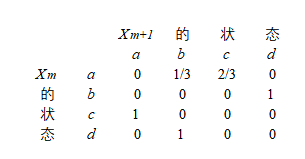以上求解转移矩阵参考此博客，详细可以了解此博客：https://blog.csdn.net/sinat_35821976/article/details/77094935

另外：求时间状态分布图 （关键字：matplotlib堆叠直方图）
import time
from tqdm import tqdm
from tqdm._tqdm import trange
import matplotlib.pyplot as plt
import numpy as np
import matplotlib
def GetPeoCount(Index):
TimeRow = dt4.iloc[:,Index:Index+1]
Act_list = ['Sleep','HouseAct','WithChild','Work','Educaton','Consumer','L.S.R','Other']
#总人数
peo_sum = 0
for x in Act_list:
#总人数：
peo_sum+= len(TimeRow[(TimeRow[Index].str.contains(x))])
Temp_list = list()
for x in Act_list:
pro= len(TimeRow[(TimeRow[Index].str.contains(x))])/peo_sum
Temp_list.append(pro)

return Temp_list

def GetList(x):
Temp_list = list()
for i in tqdm(range(144)):
num_list1 = GetPeoCount(i)
pro = GetPeoCount(i)[x]
Temp_list.append(pro)
return Temp_list

def GetMatp():
matplotlib.rcParams['font.sans-serif'] = ['SimHei']
matplotlib.rcParams['axes.unicode_minus'] = False
plt.figure(figsize=(20, 15))
temp_list = list()
for i in range(8):
temp_list.append(GetList(i))

list1 = list(temp_list)
list2 = list(temp_list)
list3 = list(temp_list)
list4 = list(temp_list)
list5 = list(temp_list)
list6 = list(temp_list)
list7 = list(temp_list)

cum1 =list(map(sum, zip(list1,list2)))
cum2 =list(map(sum, zip(list1,list2,list3)))
cum3 =list(map(sum, zip(list1,list2,list3,list4)))
cum4 =list(map(sum, zip(list1,list2,list3,list4,list5)))
cum5 =list(map(sum, zip(list1,list2,list3,list4,list5,list6)))
cum6 =list(map(sum, zip(list1,list2,list3,list4,list5,list6,list7)))

plt.bar(left=range(0, 144), height=temp_list, width=2.2, color='salmon', label="Sleep")
plt.bar(left=range(0, 144), height=temp_list, width=2.2, color='orange', label="HouseAct", bottom=temp_list)
plt.bar(left=range(0, 144), height=temp_list, width=2.2, color='gold', label="WithChild", bottom=cum1)
plt.bar(left=range(0, 144), height=temp_list, width=2.2, color='greenyellow', label="Work", bottom=cum2)
plt.bar(left=range(0, 144), height=temp_list, width=2.2, color='limegreen', label="Educaton", bottom=cum3)
plt.bar(left=range(0, 144), height=temp_list, width=2.2, color='mediumaquamarine', label="Consumer", bottom=cum4)
plt.bar(left=range(0, 144), height=temp_list, width=2.2, color='deepskyblue', label="L.S.R", bottom=cum5)
plt.bar(left=range(0, 144), height=temp_list, width=2.2, color='blue', label="Other", bottom=cum6)

plt.ylabel("百分比")
plt.xlabel("时间（单位：10分钟）")
plt.title("时间活动分布图")
plt.legend()
plt.show()

GetMatp()
注意：导入tqdm包，可视化进度条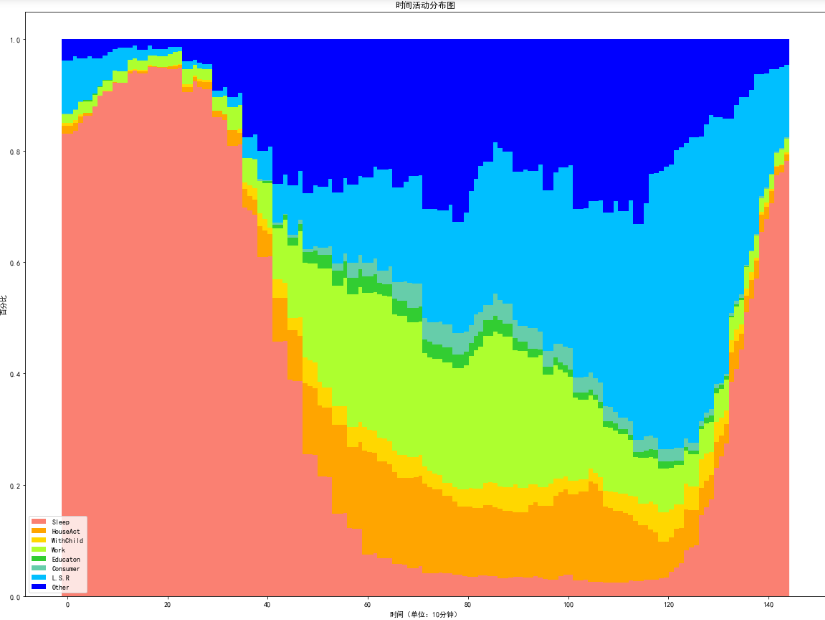展开全文pandas 数据清洗 机器学习 马尔科夫链
• 前面提到了目标的离散化，假定我们现在关心涨跌的状态（1~7），那么今天的涨跌对明天意味着什么呢？按马尔科夫的假设：下一步只受到当前步的影响。 内容 1 读取数据 获取所有股票列表 # 股票列表 #查询当前所有正常...
说明
前面提到了目标的离散化，假定我们现在关心涨跌的状态（1~7），那么今天的涨跌对明天意味着什么呢？按马尔科夫的假设：下一步只受到当前步的影响。
内容
1 读取数据
获取所有股票列表
# 股票列表
#查询当前所有正常上市交易的股票列表
stocks_df = pro.stock_basic(exchange='', list_status='L', fields='ts_code,symbol,name,area,industry,list_date')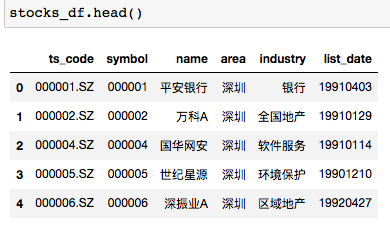获取所有指数列表
上交所
# 指数列表
index_df  = pro.index_basic(market='SSE')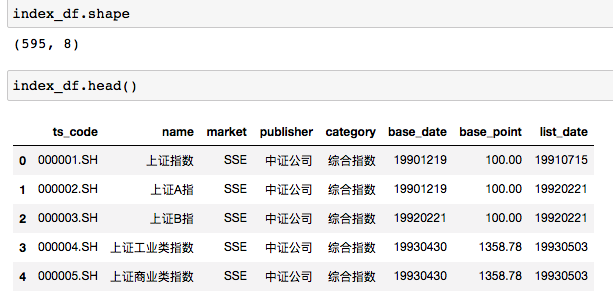2 获取股票数据
# 光明
df_gm = pro.daily(ts_code='600597.SH', start_date='20100701', end_date='20220718')
df_gm['status'] = (df_gm['pct_chg']/100).apply(fs.stock_change_status)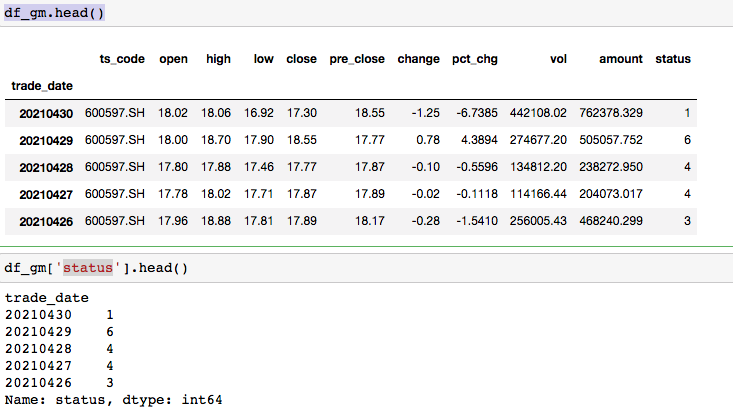输入一个离散（状态）时间序列，返回转移矩阵或者热图
# 对一个都是离散值（状态）的时间序列，计算转移矩阵
def cal_discrete_timeseries_transit_matrix(some_s ,fs, show_heat = False,missing_pct = 0.0001):
# 1 获取时间状态序列的差分
tem_df = fs.s_trans_pair(some_s)
# 2 差分序列转矩阵
trans_mat = fs.s_trans_pair2mat(tem_df)
# 3 计算转移概率矩阵
trans_mat_pct = fs.s_trans_mat2pct(trans_mat)
trans_mat_pct = trans_mat_pct.fillna(missing_pct)
if show_heat:
cols_name_list,rows_name_list, data_list = fs.make_pe_heatmap_para(trans_mat_pct)
return fs.pe_heat_map(cols_name_list,rows_name_list, data_list)
else:
return trans_mat_pct

看一下这只股票的转移矩阵
fs.cal_discrete_timeseries_transit_matrix(df_gm['status'],fs, show_heat = True)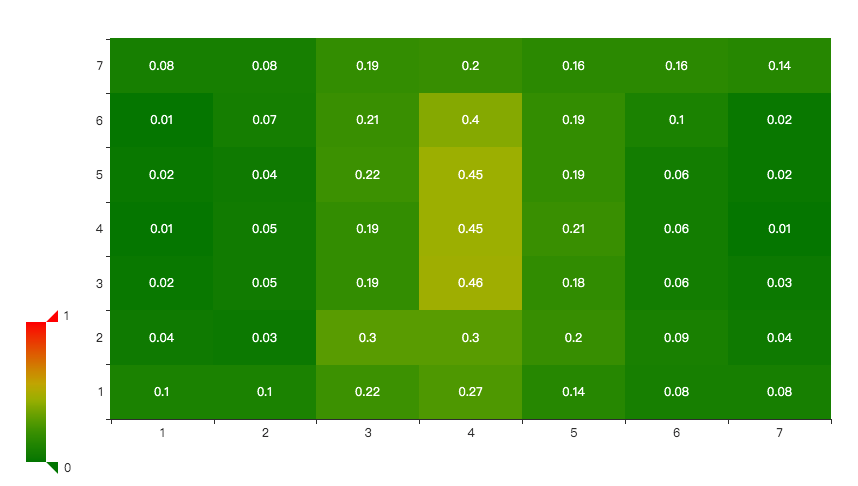2021.4.30的状态是1， 所以下一个个交易日上涨(5,6,7)的概率是30%，继续下跌的概率是24%。从概率转移矩阵上看，处于1（超级跌），2（大跌）之后行情处于更不确定的状态。以4（振荡）为中心，概率分布更加扁平。而3，4，5状态之后的变化更加确定，表现为集中在中间。在不确定的情况下，应该见好就收。
类似地，我们可以看下上证指数的变化。虽然指数的变化用1，3，5个百分点来区分比较粗糙，但是可以看规律。
fs.cal_discrete_timeseries_transit_matrix(df_sh_idx['status'],fs, show_heat = True)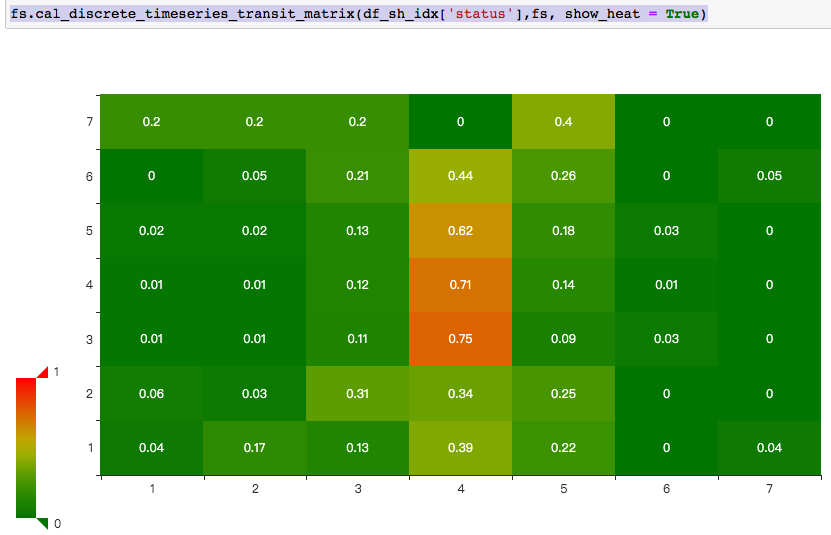总体上说，上证指数还是比较平稳的，感觉下跌状态之后倾向于继续下跌，上涨后倾向继续上涨。
3 总结
从只有一阶记忆的转移矩阵，我们可以看到一些规律。可以做出一些简单的判断。
展开全文•机器学习
• ## 一步转移概率矩阵的实现

万次阅读 多人点赞 2017-08-11 11:33:01
时间和状态都是离散的马尔科夫过程称为马尔科夫链，简称马氏链。记为 ...为马氏链在时刻m处于状态ai条件下，在时刻m+n转移到aj的转移概率。 由于链在时刻m从任何一个状态ai出发，到另一时刻...
• 马尔可夫链 注：此Java代码只实现了状态转移的个数至于概率很容易求得，具体做法可参考上面的链接或浙大概率论与数理统计第四版第十三章马尔可夫链 package legendary; import java.util.ArrayList; import java...Java 马尔可夫
• 实际分析中，往往需要知道经过一段时间后，市场趋势分析对象可能处于的状态，这就要求建立一个能反映变化规律的数学...公式中：X(k)表示趋势分析与预测对象在t=k时刻的状态向量，P表示一步转移概率矩阵， X(k+1)表...
• ## 土地利用转移矩阵制作

万次阅读 多人点赞 2019-07-11 18:03:00
随机过程具有无后效性（即在系统状态转移过程中，系统将来的状态只与现在的状态有关，而与过去的状态无关），该方法通过对各个时刻事件不同状态的初始概率及状态之间的转移关系来预测事件下一步发生的状态及其变化...
• 1 马尔可夫过程及其概率分布 2 多步转移概率的确定 3 遍历性 离散时间的马尔可夫链 连续时间的马尔可夫链人工智能 机器学习 数学
• ## 马尔科夫链与转移矩阵

万次阅读 多人点赞 2018-08-19 15:27:50
什么是转移概率矩阵(Transition Probability Matrix) 　转移概率矩阵矩阵各元素都是非负的，并且各行元素之和等于1，各元素用概率表示，在一定条件下是互相转移的，故称为转移概率矩阵。如用于市场决策时，矩阵中...
• ​国土空间规划怎么会这么忙？...通过对得到的转移矩阵进行分析，能够得到２个时相，不同的地类之间相互转化的情况，它描述了不同的土地利用的类型在不同年份发生变化的土地类别以及发生变化的位置和..arcgis
• 主要思想是不管初始状态是什么，只要状态转移矩阵不发生变化, 最终状态始终会收敛到一个固定值, 这种无记忆性叫马尔科夫属性。公式为： 2、转移概率矩阵(Transition Probability Matrix) 转移概率矩阵：矩阵各元素都...python 算法 人工智能 机器学习 大数据
• 原文地址：马尔科夫链与转移矩阵（转载）作者：怒放的生命 什么是转移概率矩阵(Transition Probability Matrix) 　转移概率矩阵：矩阵各元素都是非负的，并且各行元素之和等于1，各元素用概率表示，在一定条件下是...
• 　转移概率矩阵矩阵各元素都是非负的，并且各行元素之和等于1，各元素用概率表示，在一定条件下是互相转移的，故称为转移概率矩阵。如用于市场决策时，矩阵中的元素是市场或顾客的保留、获得或失去的概率。P(k)...
• 首先来简单介绍一下矩阵的乘法，两个矩阵能进行矩阵乘法运算的前提是：第一个矩阵（左矩阵）的列数等于第二个矩阵（右矩阵）的行数。A = （aij）是一个m*s矩阵，B = （bij）是一个s*n矩阵，则矩阵A与矩阵B的乘积是一...数学建模
• ## 土地利用转移矩阵的几种实现方法

万次阅读 多人点赞 2018-05-29 09:11:07
土地利用转移矩阵生成的几种方法 查阅相关的资料，也没有得到土地利用类型转换矩阵确切的定义，我理解为不同时间段内同一区域内土地利用类型的相互转换关系，一般用二维表来表达，从二维表中可以快速查看各个地类间...
• // /**********************************遍历结果是一步状态转移概率矩阵*******************************/ for (int s = 0; s < statusValueNum.length; s++) { // 控制数组的行数变化 s充当了状态值 ...java
• 目录马尔可夫模型马尔可夫性质转移概率计算参考资料致谢 马尔可夫模型 马尔可夫链因安德烈·马尔可夫（Andrey Markov，1856－1922）得名，是数学中具有马尔可夫性质的离散时间随机过程。该过程中，在给定当前知识或...马尔科夫链 机器学习
• 瞬时概率 瞬时概率矩阵 ...经过上面的栗子，我们已经可以通过对一个满足Markov分布的事件（无记忆的）进行状态转移图建模，还可以得出它的一步状态转移矩阵，还可以知道任一时刻各自状态发...
• 在每个步骤中，冲浪者都会从当前网页的链出网页中，随机选取一张作为下一步访问的目标。 此外，PageRank 还引入了随机的跳转操作，这意味着冲浪者不是按 Web 图的拓扑结构走下去，只是随机挑选了一张网页进行跳转 ...算法
• 马尔可夫过程按照其状态和时间参数是否连续或者离散分为三种：1.时间和状态都离散的叫做马尔科夫链，2.时间和状态都是连续的叫做马尔科夫过程，3.时间连续，状态离散的叫做连续时间的马尔科夫链。 马尔可夫过程，...
• 称为二步转移矩阵，也即由12月份的情况通过2步转移到2月份的情况。二步转移概率矩阵正好是一步转移概率矩阵的平方。一般地， k步转移概率矩阵 　正好是一步转移概率矩阵的k次方。可以证明，k步转移概率矩阵中,各...transition matrix
• 首先要做的是——写出状态表示和状态转移方程，有可能会引入k，即对于子问题的界定。——状态值dp[][]要和题目所求内容的要求符合！ 子问题——很重要，把问题转化为多个阶段的子问题。 多阶段决策过程，...
• 随机过程(1)__马尔可夫链的基本概念__马尔可夫性质__转移概率矩阵 北美高校就读统计,主要研究数理统计。空闲之时会在博客内会和大家share一些数理统计的基础知识。在这个系列的博客，我将会为大家献上随机过程的主要...算法
• ## 矩阵

2013-06-13 17:36:17
好像目前还没有这方面题目的总结。这几天连续看到四个问这类题目的人，...一个n行m列的矩阵可以乘以一个m行p列的矩阵，得到的结果是一个n行p列的矩阵，其中的第i行第j列位置上的数等于前一个矩阵第i行上的m个数与后一个C ACM...